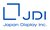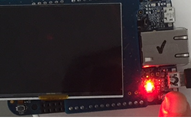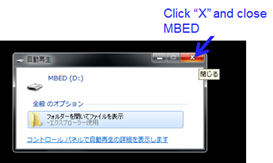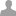We develop, design, manufacture, and sell "display" where it is necessary for the interface that deliver a lot of information at an instant and deliver it to the global market. ...

## FAQ MIP8

I will post some frequently asked questions here.

[FAQ01]the LCD(JDI MIP display) will not be displayed

When turning on the power, the red and green LEDs on the bottom board (K - 64F) will light up and the LCD will not be displayed.We assume that the program of the board changedaccidentally. Please install bin file in to the board agan. When the power supply is supplied to the board from PC , please pay attention to the followings. (1) When the demo board connects to PC to obtain the power supply, the board accesses to PC and MBED is going to open automatically. (2) When you see MBED ( MBED(D:), MBED(E:), MBED(F:), etc.) on the PC screen, click “X” and close MBED.[FAQ02] Please tell me how to ask questions such as JapanDisplayInc code of mbed site.

I would like to enter the following site (Japan DisplayInc).

https://os.mbed.com/teams/JapanDisplayInc/

Next, press the [? Ask a Quetion] button in the Community tag. Fill in the title and question contents, I'd like to contribute with "Post New Question". It is premised that it will be published to the world, JapanDisplayInc People who know the homepage can see the question. Because it is the homepage of ARM company, please understand when using.

[FAQ-03] Regarding the VCOM frequency when using EXTMODE = "L", is the following formula correct?

I understand that you invert VCOM with M1 flag, In fact, when using it in the following cases, what is the VCOM frequency? (FCLK is 2 MHz)

(1) One screen rewriting (eg 2.7 ", 4-bit mode, (400 x 4) x 240)

Number of bits= (mode select + gata line + (data number x 4 bit mode)) x 240 line + data transfar =(6 + 10 + (400 x 4)) x 240 + 16 = 387, 856 bit

Frame frequency: 2 MHz x 387, 856 bit = 1 / (2 * 10 ^ 6) x 387, 856 = 0.194 s = about 5.2 Hz Therefore, in this case the VCOM frequency is about 2.6 Hz.

(2) Area rewriting (example: 8 lines)

Number of bits=([6 + 10 + (400 x 4)) x 8 + 16 = 12,944 bit

Frame frequency = 2 MHz x 12, 944 bit = 1 / (2 * 10 ^ 6) x 12, 944 = 0.00647 s = about 154.5 Hz

Therefore, in this case the VCOM frequency is about 77.3 Hz. Since the upper limit of the VCOM specification of the specification is 70 Hz, can not rewrite 8 Line?

[FAQ-03] Line address autoincrement (increment) has no panel function. For lines to be refreshed, it is necessary to send the address of the line before sending the pixel data of that line. Is it necessary to send a new address again when updating the next line, and before sending pixel data?

This product can not be rewritten by specifying the address of multiple lines to be refreshed in advance. To refresh the display you need to send the line address and data.

####Mbed_developer JDI wrote:

[FAQ-03] Regarding the VCOM frequency when using EXTMODE = "L", is the following formula correct?

I understand that you invert VCOM with M1 flag, In fact, when using it in the following cases, what is the VCOM frequency? (FCLK is 2 MHz)

(1) One screen rewriting (eg 2.7 ", 4-bit mode, (400 x 4) x 240)

Number of bits= (mode select + gata line + (data number x 4 bit mode)) x 240 line + data transfar =(6 + 10 + (400 x 4)) x 240 + 16 = 387, 856 bit

Frame frequency: 2 MHz x 387, 856 bit = 1 / (2 * 10 ^ 6) x 387, 856 = 0.194 s = about 5.2 Hz Therefore, in this case the VCOM frequency is about 2.6 Hz.

(2) Area rewriting (example: 8 lines)

Number of bits=([6 + 10 + (400 x 4)) x 8 + 16 = 12,944 bit

Frame frequency = 2 MHz x 12, 944 bit = 1 / (2 * 10 ^ 6) x 12, 944 = 0.00647 s = about 154.5 Hz

Therefore, in this case the VCOM frequency is about 77.3 Hz. Since the upper limit of the VCOM specification of the specification is 70 Hz, can not rewrite 8 Line?